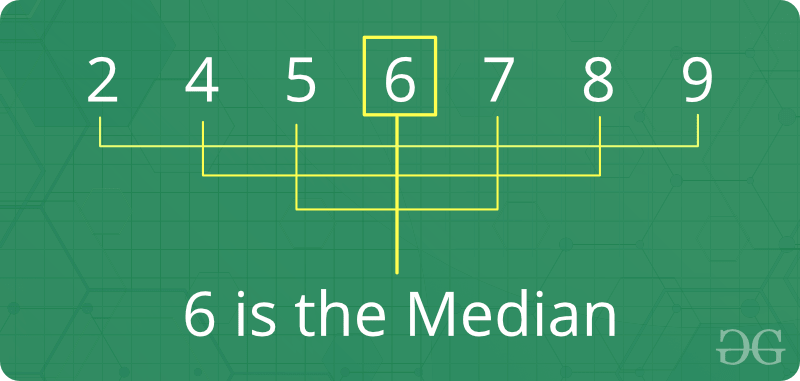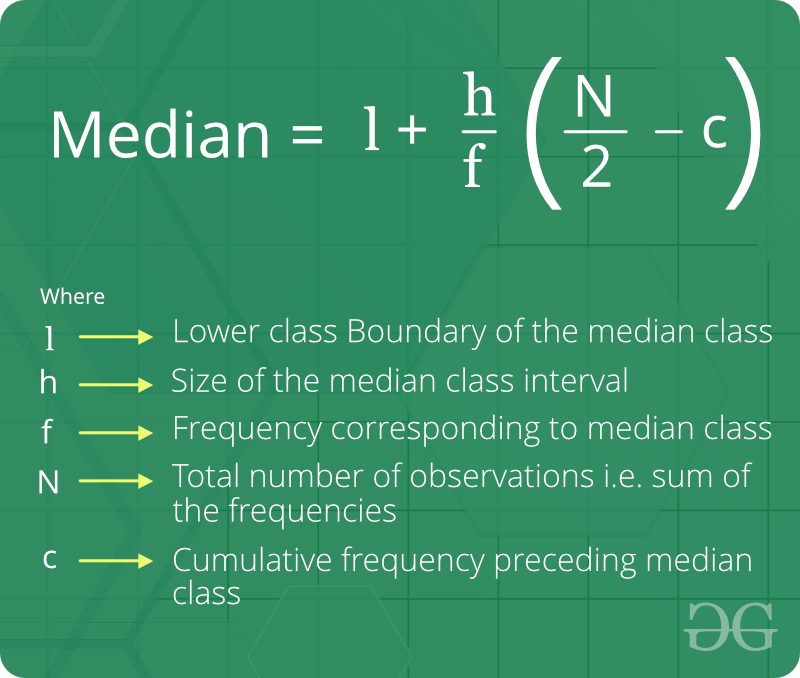# Median

Median is the middle value of a set of data. To determine the median value in a sequence of numbers, the numbers must first be arranged in ascending order.

• If there is an odd amount of numbers, the median value is the number that is in the middle, with the same amount of numbers below and above.
• If there is an even amount of numbers in the list, the median is the average of the two middle values.1. Median is joined by the mean and the mode to create a grouping called measures of central tendency.
2. Median is an important measure (compared to mean) for distorted data, because median is not so easily distorted. For example, median of {1, 2, 2, 5, 100) is 2 and mean is 22.
3. If user add a constant to every value, the mean and median increase by the same constant.
4. If user multiply every value by a constant, the mean and the median will also be multiplied by that constant.

Formula of Median of ungrouped data :Formula of Median of grouped data :How to find a median of an unsorted array ?

Naive solution:
Given n size unsorted array, find its median.

Median of a sorted array of size n is defined as below :

It is middle element when n is odd and average of middle two elements when n is even. Since the array is not sorted here, we sort the array first, then apply above formula.

Examples:

```Input  : {1, 3, 4, 2, 6, 5, 8, 7}
Output : Median = 4.5

Since number of elements are even, median
is average of 4th and 5th largest elements.
which means (4 + 5)/2 = 4.5

Input  : {4, 4, 4, 4, 4}
Output : Median = 4
```

Below is the code implementation:

## C++

 `// CPP program to find median ` `#include ` `using` `namespace` `std; ` ` `  `// Function for calculating median ` `double` `findMedian(``int` `a[], ``int` `n) ` `{ ` `    ``// First we sort the array ` `    ``sort(a, a + n); ` ` `  `    ``// check for even case ` `    ``if` `(n % 2 != 0) ` `        ``return` `(``double``)a[n / 2]; ` ` `  `    ``return` `(``double``)(a[(n - 1) / 2] + a[n / 2]) / 2.0; ` `} ` ` `  `// Driver program ` `int` `main() ` `{ ` `    ``int` `a[] = { 1, 3, 4, 2, 7, 5, 8, 6 }; ` `    ``int` `n = ``sizeof``(a) / ``sizeof``(a); ` `    ``cout << ``"Median = "` `<< findMedian(a, n) << endl; ` `    ``return` `0; ` `} `

## Java

 `// Java program to find median ` `import` `java.util.*; ` ` `  `class` `GFG { ` ` `  `    ``// Function for calculating median ` `    ``public` `static` `double` `findMedian(``int` `a[], ``int` `n) ` `    ``{ ` `        ``// First we sort the array ` `        ``Arrays.sort(a); ` ` `  `        ``// check for even case ` `        ``if` `(n % ``2` `!= ``0``) ` `            ``return` `(``double``)a[n / ``2``]; ` ` `  `        ``return` `(``double``)(a[(n - ``1``) / ``2``] + a[n / ``2``]) / ``2.0``; ` `    ``} ` ` `  `    ``// Driver program ` `    ``public` `static` `void` `main(String args[]) ` `    ``{ ` `        ``int` `a[] = { ``1``, ``3``, ``4``, ``2``, ``7``, ``5``, ``8``, ``6` `}; ` `        ``int` `n = a.length; ` `        ``System.out.println(``"Median = "` `+ findMedian(a, n)); ` `    ``} ` `} `

## Python3

 `# Python3 program to find median ` ` `  `# Function for calculating median ` `def` `findMedian(a, n): ` ` `  `    ``# First we sort the array ` `    ``sorted``(a) ` ` `  `    ``# check for even case ` `    ``if` `n ``%` `2` `!``=` `0``: ` `        ``return` `float``(a[n ``/``/` `2``]) ` `     `  `    ``return` `float``((a[``int``((n``-``1``)``/``2``)] ``+` `                  ``a[``int``(n ``/` `2``)])``/``2.0``) ` ` `  `# Driver program ` `a ``=` `[ ``1``, ``3``, ``4``, ``2``, ``7``, ``5``, ``8``, ``6` `] ` `n ``=` `len``(a) ` `print``(``"Median ="``, findMedian(a, n)) `

## C#

 `// C# program to find median ` `using` `System; ` ` `  `class` `GFG { ` ` `  `    ``// Function for ` `    ``// calculating median ` `    ``public` `static` `double` `findMedian(``int``[] a, ``int` `n) ` `    ``{ ` `        ``// First we sort ` `        ``// the array ` `        ``Array.Sort(a); ` ` `  `        ``// check for ` `        ``// even case ` `        ``if` `(n % 2 != 0) ` `            ``return` `(``double``)a[n / 2]; ` ` `  `        ``return` `(``double``)(a[(n - 1) / 2] + a[n / 2]) / 2.0; ` `    ``} ` ` `  `    ``// Driver Code ` `    ``public` `static` `void` `Main() ` `    ``{ ` `        ``int``[] a = { 1, 3, 4, 2, ` `                    ``7, 5, 8, 6 }; ` `        ``int` `n = a.Length; ` `        ``Console.Write(``"Median = "` `+ findMedian(a, n) + ``"\n"``); ` `    ``} ` `} `

## PHP

 ` `

Output:

```Median = 4.5
```

Time Complexity to find median = O(n Log n) as we need to sort the array first. Note that we can find median in O(n) time using methods discussed here and here.

Basic Program related to Median:

More problems related to Median:

Recent Articles on Median!

Attention reader! Don’t stop learning now. Get hold of all the important DSA concepts with the DSA Self Paced Course at a student-friendly price and become industry ready.

My Personal Notes arrow_drop_upIf you like GeeksforGeeks and would like to contribute, you can also write an article using contribute.geeksforgeeks.org or mail your article to contribute@geeksforgeeks.org. See your article appearing on the GeeksforGeeks main page and help other Geeks.

Please Improve this article if you find anything incorrect by clicking on the "Improve Article" button below.

Improved By : ananth2four

Article Tags :
Practice Tags :

4

Please write to us at contribute@geeksforgeeks.org to report any issue with the above content.